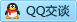MathWorks – MATLAB 加强学术研究，为教育行业提供无限扩展和合作机会

今天，MathWorks宣布扩展对 MATLAB Parallel Server 的访问，以帮助加快学术研究。现在，拥有 MathWorks Campus-Wide License 全校授权许可（包括 MATLAB Parallel Server）的学术机构的所有研究人员和学生都有无限访问权，可将 MATLAB 程序和 Simulink 仿真扩展到集群和云。全新的灵活使用策略还延伸到来自其他学术机构的客座教授和研究人员，从而实现合作。

MATLAB Parallel Server 可无缝使用额外的计算机资源来加快研究速度。现在，在大学拥有的集群上或 Amazon Web Services 和 Microsoft Azure 之类的云上，全校的教职员工、研究人员和学生都可以运行不限数量的并发 MATLAB 计算引擎（称为 MATLAB worker）。MATLAB Parallel Server 可通过熟悉的 MATLAB 环境访问集群资源、无需更改算法便可在多台机器上运行，同时有助于访问云集群或本地高性能计算（HPC）资源。

扩展访问还可向来自其他机构的学术研究人员、学生和教职员工提供 MATLAB 和 Simulink 的访问权限，从而灵活地开展合作。现在，这些学者可以在授权的校园计算机和大学 HPC 集群上访问 MATLAB，从事非商业工作。这有助于支持机构间的合作研究项目，提供对可能存储公用数据的 HPC 基础架构的访问，无需再繁琐地检查合作者是否具有 MATLAB 许可证。

“研究是各行各业创新的基石，随着这些项目变得更加复杂，研究人员需要进行扩展和合作，从而真正获得成功。”MathWorks 的 HPC 营销负责人 Silvina Grad-Freilich 说，“我们相信，提供无限扩展和简化合作，有助于我们满足不断增长的并行计算需求，提供当今研究项目需要的联合工作机会。”

针对所有版本的 MATLAB Parallel Server，包括名为 MATLAB Distributed Computing Server的早期版本的产品，现均提供全新的扩展和合作访问。

全年征稿 / 资讯合作